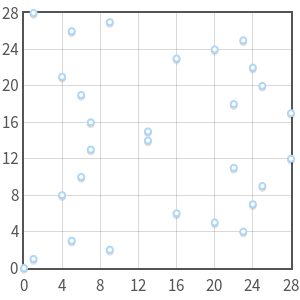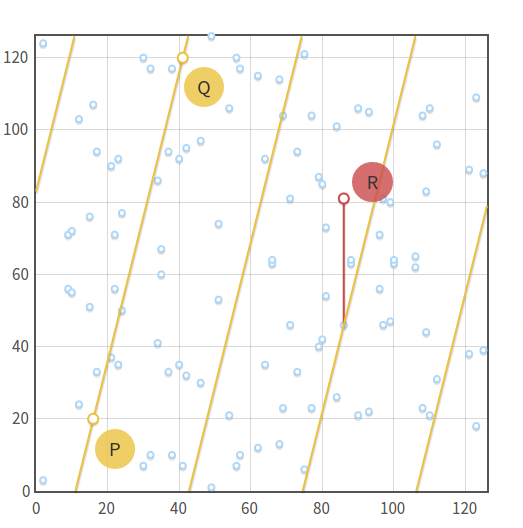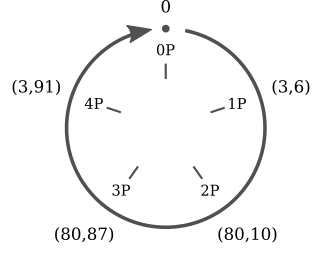# 椭圆曲线加密：有限域和离散对数

## p的整数模域(The field of integers modulo p)

• 加法• 减法• 乘法• 相反加• 特别地:• 相反幂• 特别地：## 模p的除法（division modulo p）

def extended_euclidean_algorithm(a, b):
"""
Returns a three-tuple (gcd, x, y) such that
a * x + b * y == gcd, where gcd is the greatest
common divisor of a and b.

This function implements the extended Euclidean
algorithm and runs in O(log b) in the worst case.
"""
s, old_s = 0, 1
t, old_t = 1, 0
r, old_r = b, a

while r != 0:
quotient = old_r // r
old_r, r = r, old_r - quotient * r
old_s, s = s, old_s - quotient * s
old_t, t = t, old_t - quotient * t

return old_r, old_s, old_t

def inverse_of(n, p):
"""
Returns the multiplicative inverse of
n modulo p.

This function returns an integer m such that
(n * m) % p == 1.
"""
gcd, x, y = extended_euclidean_algorithm(n, p)
assert (n * x + p * y) % p == gcd

if gcd != 1:
# Either n is 0, or p is not a prime number.
raise ValueError(
'{} has no multiplicative inverse '
'modulo {}'.format(n, p))
else:
return x % p


##上的椭圆曲线(Elliptic curves in)•(这个由唯一元素(identity)定义可得)。
• 给定一个非零点, 其相反数是一个拥有相同的横坐标但是具有相反的纵坐标的点。或者如果你愿意的话，例如，一个曲线在域上，有这样的一点,那么其相反点• 同样的，（从相反元素的定义可得）

## 代数和(Algebraic sum)## 标量乘法和循环子群(Scalar multiplication and cyclic subgroups

)上的椭圆曲线的乘法是具有一些有趣的属性。我们拿曲线和其上的点。然后我们计算一下P的乘法。倍数只有五个独立的点这是一个不断循环的环。从中可以非常简单地看出在椭圆曲线上的标量乘法和模代数运算中加法的相似性。

• 0P=0
• 1P=(3,6)
• 2P=(80,10)
• 3P=(80,87)
• 4P=(3,91)
• 5P=0
• 6P=(3,6)
• 7P=(80,10)
• 8P=(80,87)
• 9P=(3,91)

1. P的倍数只有五种，其他在椭圆曲线上的点不会出现。
2. 他们是“重复的环”。

• 5kP=0
• (5k+1)P=P
• (5k+2)P=2P
• (5k+3)P=3P
• (5k+4)P=4P“子群”是另一个群的子集，一个“循环子群”是一个所有的元素会循环重现的子群，就像我们前面展示的例子一样。那么这个点P就被称为这个循环子群的生成者(generator)或者基点(base point)。

## 子群的阶(Subgroup order)

• 目前，我们定义了一个群的阶就是这个群中点的个数。这个定义还是有效的，但是在循环子群中，我们需要给一个新的等价定义：点P的阶是一个最下的能够让等式nP=0的正整数。实际上，如果你看到前面的例子，我们的子群包括5个点，而且我们有• P的阶在Lagrange’s theorem中有详细阐述，主要的意思是：子群的阶是父群的阶的一个因数，换句话说，如果一个椭圆曲线包括N个点，那么它的一个子群就包括n个点，而且，n是N的一个因数。

1. 利用Schoof算法计算椭圆曲线的阶N
2. 找到所有N的因数
3. 对于所有N的的因数n，计算nP
4. 找到最小能够让成立的n，这就是子群的阶。## 找到一个基点(Finding a base point)1. 计算椭圆曲线的阶N
2. 选择一个合适的子群阶n,为了算法能够工作，这个数必须是一个素数且必须是N的一个因数。
3. 计算辅因子4. 在曲线上选择一个随意的点P
5. 计算6. 如果G是0,那么重复第4步。直到我们找到一个子群的基点 能够让这个子群的阶为n以及其辅因子为h。

#EOF